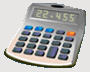DosageHelp.com Helping Nursing Students Prepare for Medication Exams By Explaining Dosage Calculations

# Mass/Time - IV mL Rate Questions

Give an order in quantity of mass per time, determine the necessary IV flow rate in mL/hr based on the given mass per volume. These types of problems are often used in critical care nursing.

Formula:
 Ordered Per Hour Have
x Volume (mL)  =  Y (Flow Rate in mL/hr)

Example: Give patient 500 mg of dopamine in 250 mL of D5W to infuse at 20 mg/hr. Calculate the flow rate in mL/hr.
 Ordered Per Hour Have
x Volume (mL)  =  Y (Flow Rate in mL/hr)
 20 mg/hr 500 mg
x 250 mL  =  10 mL/hr

Example: Aggrastat at 12.5 mg in 250 mL is prescribed to be infused at a rate of 6 mcg/kg/hr in a patient who weighs 100 kg. At what flow rate in mL/hr will you set the pump?
 Ordered Per Hour Have
x Volume (mL)  =  Y (Flow Rate in mL/hr)
• The first step is to convert the order per time to the amount required for this particular patient. This is a Dosage By Weight Question. 100 kg is the weight in kg and 6 mcg/kg/hr is a dosage in terms of kg.
 Weight in Kg * Dosage Per Kg =  Y (Required Dosage)
 100 kg * 6 mcg/kg/hr =  600 mcg/hr
Convert 600 mcg/hr to mg/hr.
• mcg → mg → g → kg    ( ÷ by 1,000 )
• 600 ÷ 1,000 = 0.6 mg/hr
 0.6 mg/hr 12.5 mg
x 250 mL  =  12 mL/hr

Congratulations: You have completed this tutorial. Click on the button below to go to sample questions and practice your new skills. You can also click here to sign up for the dosage calculation question of the day or click here to tell a friend about DosageHelp.com. Good luck future nurses!

Home Dosage Question Steps Unit Conversion Mass for Mass Mass/Liquid For Liquid IV Terms & Abbreviations Amount in IV Fluid Volume/Time - IV mL Rate Volume/Time - IV Drop Rate Fluid Maintenance Dosage By Weight Mass/Time - IV mL Rate Practice Questions Question of the Day Tell a Friend Print a Flyer